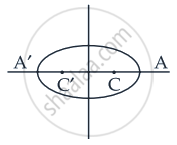# An ellipse is described by using an endless string which is passed over two pins. If the axes are 6 cm and 4 cm, the length of the string and distance between the pins are ______. - Mathematics

Fill in the Blanks

An ellipse is described by using an endless string which is passed over two pins. If the axes are 6 cm and 4 cm, the length of the string and distance between the pins are ______.

#### Solution

An ellipse is described by using an endless string which is passed over two pins. If the axes are 6 cm and 4 cm, the length of the string and distance between the pins are 6 + 2sqrt(5) cm and 2sqrt(5) cm.

Explanation:Let equation of ellipse is x^2/a^2 + y^2/b^2 = 1

Here 2a = 6 ⇒ a = 3

And 2b = 4 ⇒ b = 2

We know that c2 = a2 – b2

= (3)2 – (2)2

= 9 – 4

= 5

c = sqrt(5)

We have e = c/a

⇒ e = sqrt(5)/3

Length of string = 2a + 2ae = 2a(1 + e)

= 6(1 + sqrt(5)/3)

= (6(3 + sqrt(5)))/3

= 6 + 2sqrt(5)

Distance between the pins = CC'

= 2ae

= 2 xx 3 xx sqrt(5)/3

= 2sqrt(5)

Concept: Ellipse - Standard Equations of an Ellipse
Is there an error in this question or solution?

#### APPEARS IN

NCERT Mathematics Exemplar Class 11
Chapter 11 Conic Sections
Exercise | Q 43 | Page 205